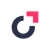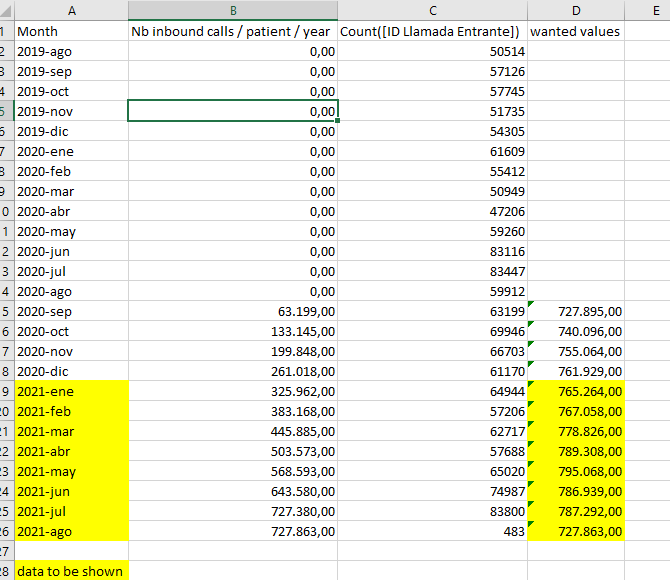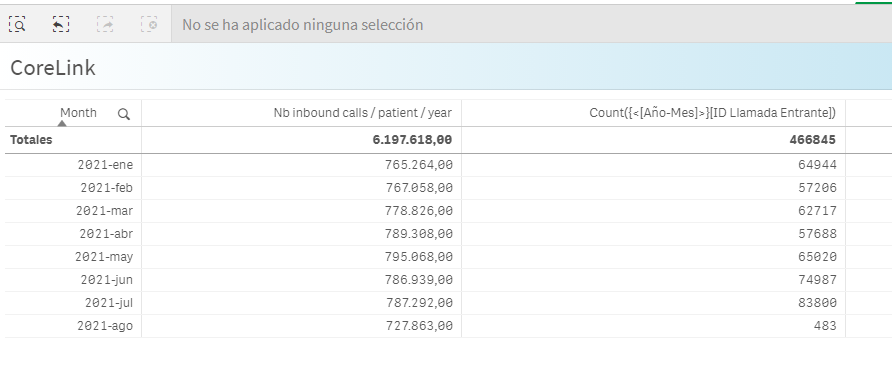# App Development

cancel
Showing results for
Did you mean:Creator

## Avoid filter on agregate funtcion

Hi Community!

We're facing an undesirable behavior in an agregation funcion working with filters.

I'll try to explain myself with screenshots and expresions.

We have a table with YearMonth dimension  and an aggr expresion:

Sum({<\$(vSetRolling12Modifier)>}Aggr(Rangesum(Above(count({<\$(vSetRolling12Modifier)>}[ID Llamada Entrante]),0,12)), ([Año-Mes], (Numeric, Ascending)) ))

with this expression we want to show, for every yearmonth, the number of "ID Llamada Entrante" in that month and previous 11  (for 2021-june, the amount of 2020-july -> 2021-june; for 2021-may, the amount of 2020-june -> 2021-may)

Where \$(vSetRolling12Modifier) =

[Date]=,[Day]=,[Weekday]=,[Year]=,[Month]=,[Quarter]=,[YearMonth]=,
[_MonthSerial] = {">=\$(=Max({1<\$(_f_DateIsFuture)={0},_Qvc.DummyField=\$(_Qvc.Calendar.v.SelectedFieldList_)>} [_MonthSerial]) - 11)<=\$(=Max({1<\$(_f_DateIsFuture)={0},_Qvc.DummyField=\$(_Qvc.Calendar.v.SelectedFieldList_)>} [_MonthSerial]))"}

We use vSetRolling12Modifier to avoid calendar filters and to take into account the previous 12 months

It works fine when we don't apply filter (data has been validated):But when we apply a YearMonth filter, previous months data changes, wich is no correct.Can someone help us to reach the correct expression?

Regards,

Alonso Torres.

1 Solution

Accepted SolutionsCreator
Author

Hi all!

Finally we had solved our expression problem. Briefly, We did tree modifications:

• we modified the limit of data load to load curren year and two year before. (now, 2019 to 2021)
• we also modified year-month dimension on table to limit showed yearmonths (now, previous year - january to current year-month). We add Year dimension an limited it.
• year dimension is  =IF(year([Year-Month])>=Year(today())-1,[Year],null())
• finally, we modified the expression to rolling over all year to current yearmonth maintaining year-month ignoring in SetAnalysis.
• Expression now is: Sum({<DateField={">=\$(=yearstart(max(DateField),-2))<=\$(=max(DateField))"},Month=,Year=,[Year-Month]=>}Aggr(Rangesum(Above(count({<DateField={">=\$(=yearstart(max(DateField),-2))<=\$(=max(DateField))"},Month=,Year=,[Year-Month]=>}[ID Llamada Entrante]),0,12)), ([Year-Month], (Numeric, Ascending)) ))

Those modifications makes our table to show desired data.

Best regards,

Alonso Torres.

7 RepliesMVP

I think you may need the modifier inside the Aggr() as well.

Sum({<\$(vSetRolling12Modifier)>}Aggr({<\$(vSetRolling12Modifier)>}Rangesum(Above(count({<\$(vSetRolling12Modifier)>}[ID Llamada Entrante]),0,12)), ([Año-Mes], (Numeric, Ascending)) ))

-RobCreator
Author

Hi Rob,

Thanks for your post, but it didn't work.

I think maybe the modifier isn't correct for what we want.

I attach here a simplified copy of our app for you, community, to test it.

What we want is to see in "Nb inbound calls..." column the sum of count(ID Llamada Entrante) for the month plus previous 11 months. But showing only current year.

I.E., for 2021-jun, first column must show the count of ID Llamada Entrante since 2020-jul to 2021-jun, regardless data filter.

This image shows what we have and what we want:Best regards,

Alonso TorresMVP

@Gestion-PSD  you need to add the modifier in other expression as well. Then go to add-on and uncheck include zero values optionCreator
Author

Thanks, Kushal, but this isn't the aim of the post.

In the real app, [ID Llamada Entrante] column doesn't appear. I put it in the image and exaple app to show the values that must be aggregated in [Nb inbound calls] column.

We want to know how to rewrite [Nb inbound calls] expression to be independent of date filtering.

Regards,

Alonso Torres.MVP

@Gestion-PSD  still not clear.. So when you don't have any filter selection, you want to show last 12 months period and each period will have values aggregated for last 12 months? If yes, then any other period filter selection should not affect this view?Creator
Author

We want an expresion (I understand that that expression must be an aggr one) that shows previous 12 months count for a field for every shown year-month.

But with some "special" features

• this aggr expression must be independent of year-month filtering
• The table only shows months of current year.

These are correct values we expect (using Sum({<[Año-Mes]=>}Aggr(Rangesum(Above(count({<[Año-Mes]=>}[ID Llamada Entrante]),0,12)), ([Año-Mes], (Numeric, Ascending)) )) and IF(year([Año-Mes])=Year(today()),[Año-Mes],null()) in dimension:But it has a problem. If we filter by [Año-Mes]  = 2021-Jun in order to show only Jan to Jun data, table doesn't change.

So, we need an expression with a rolling modifier (i suppose) that can show theese values but only for 2021-Jan to year-month_used_as_filter. I mean, I we apply [Año-Mes] = 2021-Jun filter, table must show exatly first 6 rows, without any changes in column values.

The expression we are using doesn't have this behavior, because its values change when we use year-month filter.

Best regards,

Alonso Torres.Creator
Author

Hi all!

Finally we had solved our expression problem. Briefly, We did tree modifications:

• we modified the limit of data load to load curren year and two year before. (now, 2019 to 2021)
• we also modified year-month dimension on table to limit showed yearmonths (now, previous year - january to current year-month). We add Year dimension an limited it.
• year dimension is  =IF(year([Year-Month])>=Year(today())-1,[Year],null())
• finally, we modified the expression to rolling over all year to current yearmonth maintaining year-month ignoring in SetAnalysis.
• Expression now is: Sum({<DateField={">=\$(=yearstart(max(DateField),-2))<=\$(=max(DateField))"},Month=,Year=,[Year-Month]=>}Aggr(Rangesum(Above(count({<DateField={">=\$(=yearstart(max(DateField),-2))<=\$(=max(DateField))"},Month=,Year=,[Year-Month]=>}[ID Llamada Entrante]),0,12)), ([Year-Month], (Numeric, Ascending)) ))

Those modifications makes our table to show desired data.